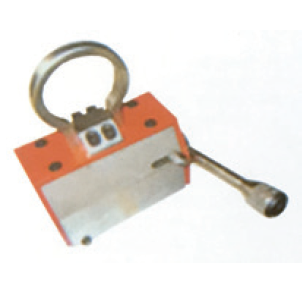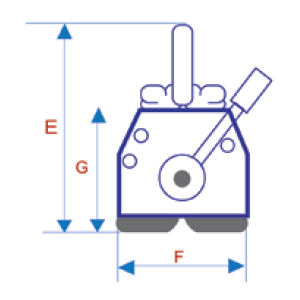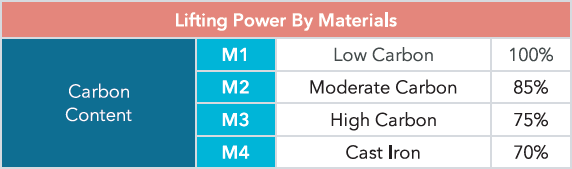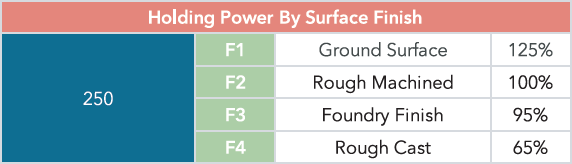## TH-LTC Permanent Lifting MagnetHigh-performance magnetic materials production, volumes is smaller, weight is lighter, holding force is greater. Unique magnetic circuit design, remanence is almost zero.
The safety factor is high, maximum pull-off force is 3.5 times than the rated lifting.
Switch handle has a security button, one-handed operation, safe and convenient.
" V " - shaped groove is on the underside of the lifter, lifting the round steel or steel plate.
No need electricity, the magnetic holding force is timeless.
Widely used in the steel handling, the formation mechanical parts as well as the installation of a variety of abrasive handling.Calculation Example:

LT-600, rated lifting power is 600 kg, the formula for calculating range of lifting capacity is: T x F x M x G x Capacity.

LT-600, for example, T=25 mm, F=F3, M=S45C Hardened and G=0.5 mm, Check the above table and list, T=85%, F=90%, M=75%, G=80%, the lifting capacity is: 85% x 90% x 75% x 80% x 600= 155 kg.

#### TH-LTC Permanent Lifting Magnet